# QUESTION 10 Find the triple scalar product (u x v). w of the vectors u =...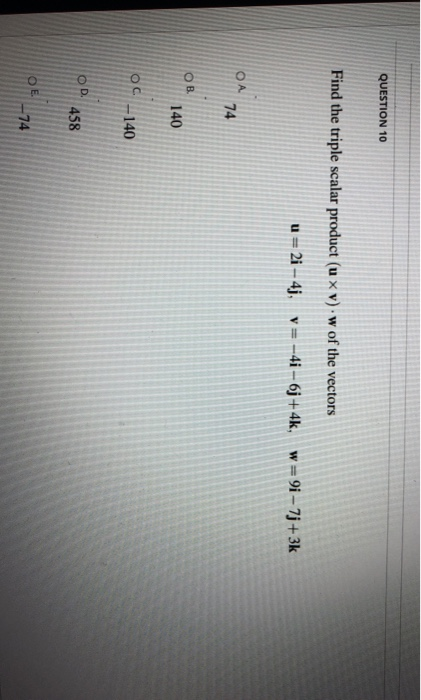QUESTION 10 Find the triple scalar product (u x v). w of the vectors u = 2i - 4j, v= -4i - 6j + 4k, w=9i - 7j+3k ОА 74 OB 140 OC-140 OD 458 ОЕ 74

First take cross product, make determinant using u and v, write the coefficients of these vectors in matrix. And find determinant.

Now find dot product of the determinant vector and w vector.

For dot product directly multiply coefficients of vectors and add it.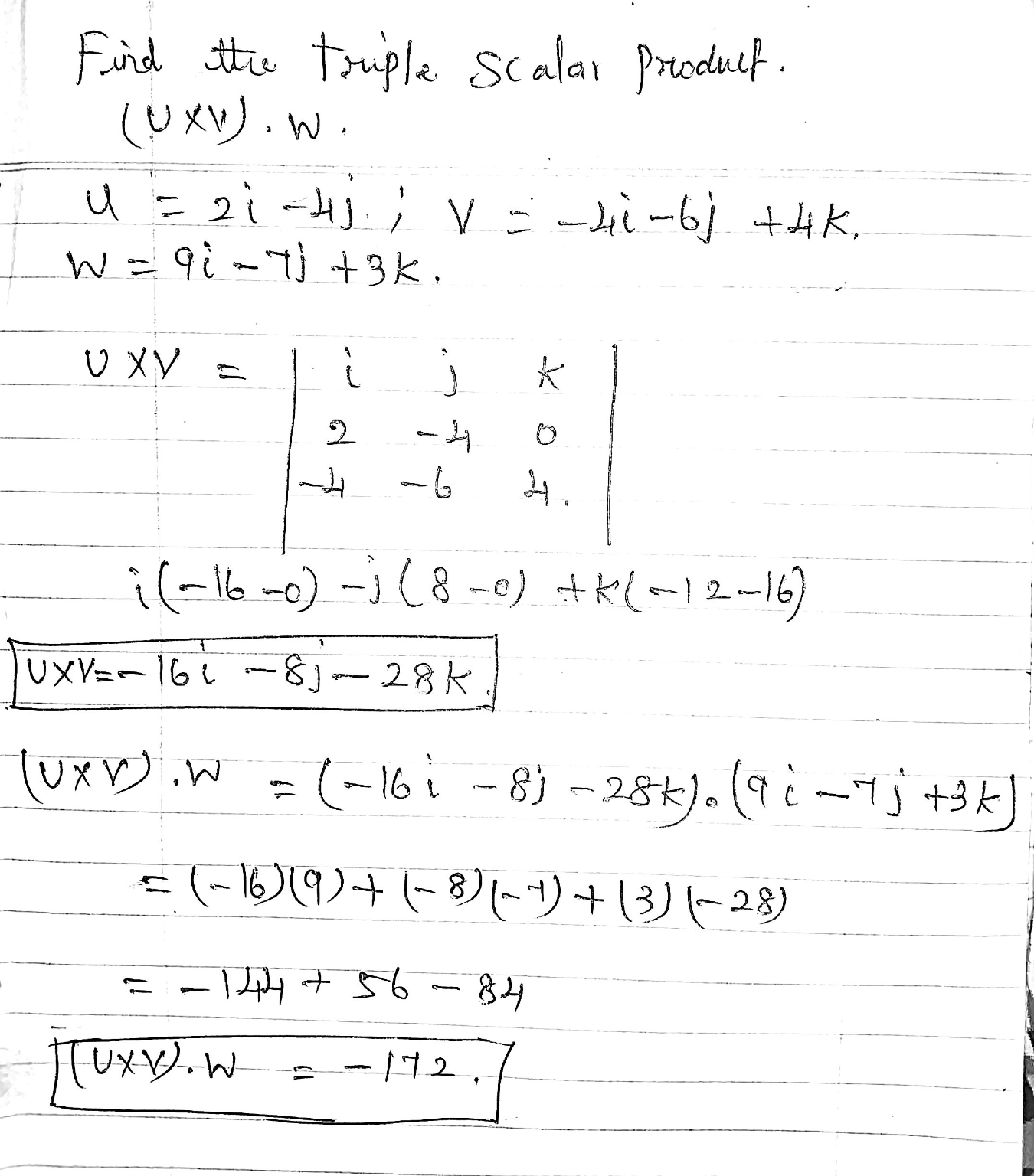##### Add Answer of: QUESTION 10 Find the triple scalar product (u x v). w of the vectors u =...
Similar Homework Help Questions
• ### Find u. (v * w). This quantity is called the triple scalar product of u, v,...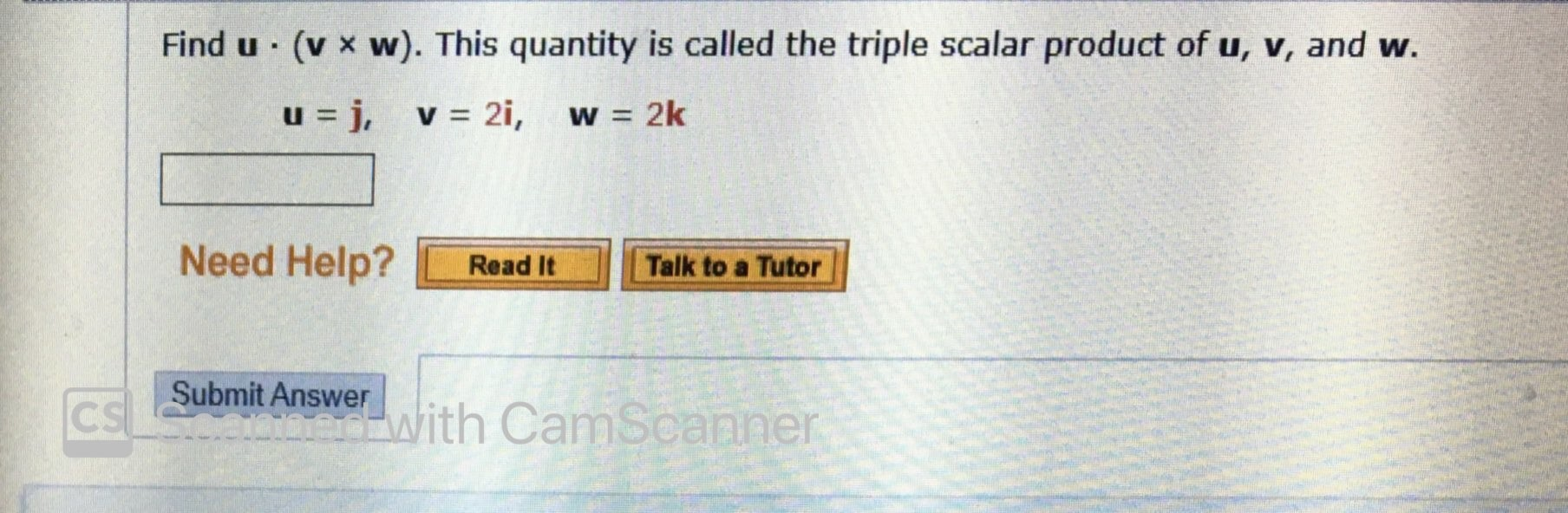Find u. (v * w). This quantity is called the triple scalar product of u, v, and w. u=j, v = 2i, w = 2k Need Help? Read It Talk to a Tutor CS Submit Answer With CamScanner onit Answer with

• ### QUESTION 18 Find the Jacobian 2(x,y) using x = 7ucosh(Sv), y = 7usinh(8v). Ə(u, v) ОА...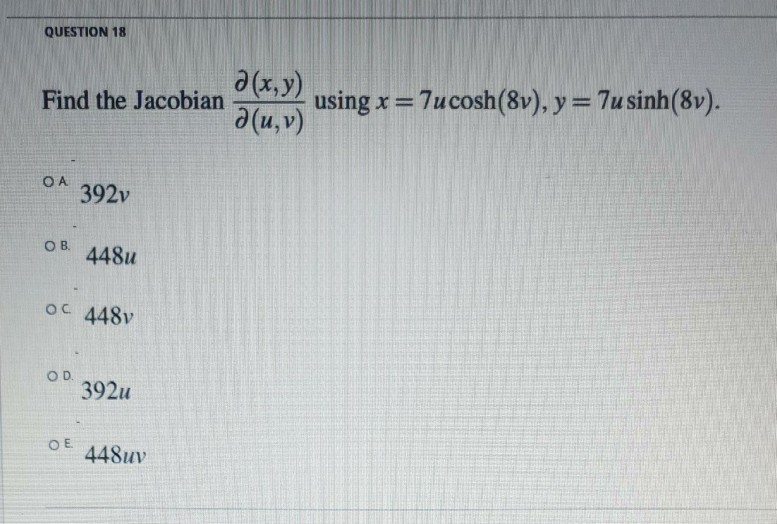QUESTION 18 Find the Jacobian 2(x,y) using x = 7ucosh(Sv), y = 7usinh(8v). Ə(u, v) ОА 392v OB 448u OC 448v OD 392u ОЕ 448uv

• ### Thank you Find u. (w). This quantity is called the triple scalar product of u, v,...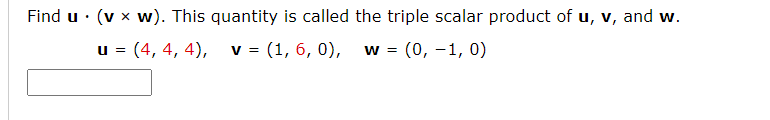Thank you Find u. (w). This quantity is called the triple scalar product of u, v, and w. u = (4, 4, 4), v = (1, 6, 0), (0, -1,0) W = Let T: R3 R3 be a linear transformation such that T(1, 1, 1) = (4,0, -1), T(0, -1, 2) = (-5,2, -1), and T(1, 0, 1) = (1, 1, 0). Find the indicated image. T(2, -1, 1) T(2, -1, 1) = Let T be a linear transformation from...

• ### 4. Determine the scalar triple product of the vectors: 3i2k and c = 3i+ j +...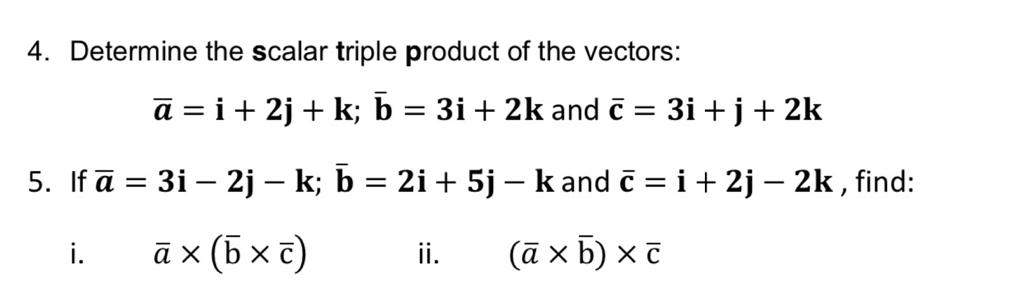4. Determine the scalar triple product of the vectors: 3i2k and c = 3i+ j + 2k a i2jk; b 5. Ifa 3i 2j - k; b = 2i +5j - kand C i + 2j - 2k, find: ax (b x c) (ax b) x t i. ii 4. Determine the scalar triple product of the vectors: 3i2k and c = 3i+ j + 2k a i2jk; b 5. Ifa 3i 2j - k; b = 2i +5j -...

• ### Find a so that the vectors v = i + aj and w = 41 -...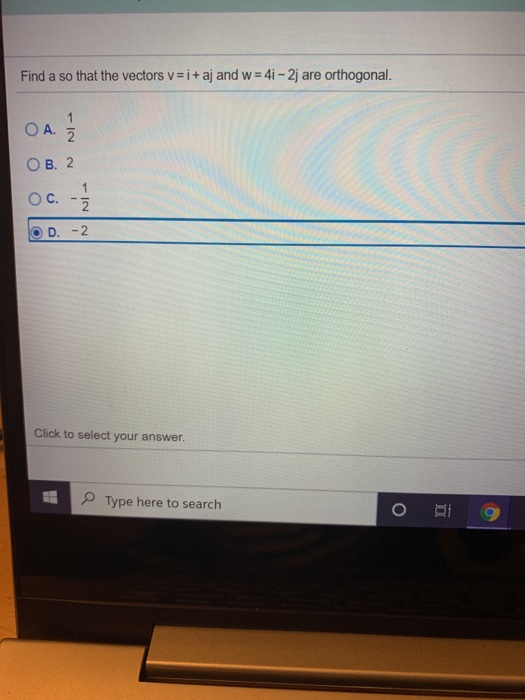Find a so that the vectors v = i + aj and w = 41 - 2j are orthogonal. 1 ОА. 2 OB. 2 OC. IN D. -2 Click to select your answer. Type here to search ві

• ### Given vectors u and v, find (a) 7u (b) 7u+6v (c) v-hu. u=9i, v = 3i...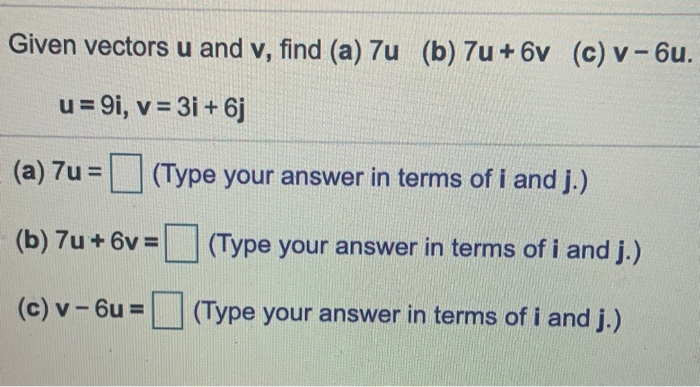Given vectors u and v, find (a) 7u (b) 7u+6v (c) v-hu. u=9i, v = 3i + 6j (a) 7u= (Type your answer in terms of i and J.) (b) 7u + 6 = (Type your answer in terms of i and j.) (c) v-6u = (Type your answer in terms of i and j.) Use the figure to evaluate a+b, a-b, and -a.

• ### use the vectors v=-2i + j and w=4i -3j to find the following 4v – 3w...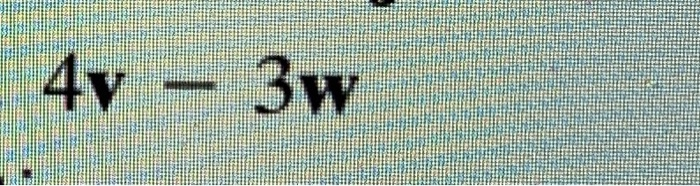use the vectors v=-2i + j and w=4i -3j to find the following 4v – 3w .

• ### Let V be the set of vectors shown below. VE :x>0, a. If u and are...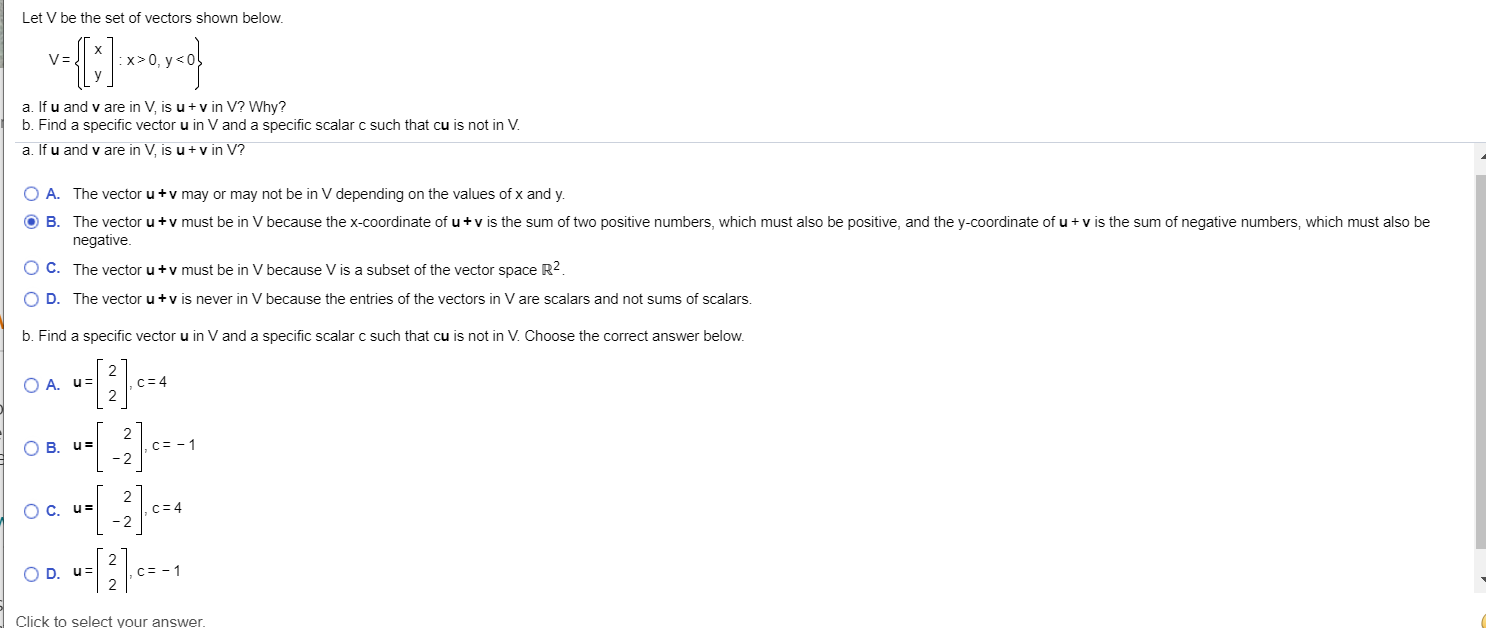Let V be the set of vectors shown below. VE :x>0, a. If u and are in V, is u +v in V? Why? b. Find a specific vector u in V and a specific scalar c such that cu is not in V. a. If u and v are in V, is u + v in V? O A. The vector u + v may or may not be in V depending on the values of x and y....

• ### Let V be the set of vectors shown below. V= Ox>0, y>0 a. If u and...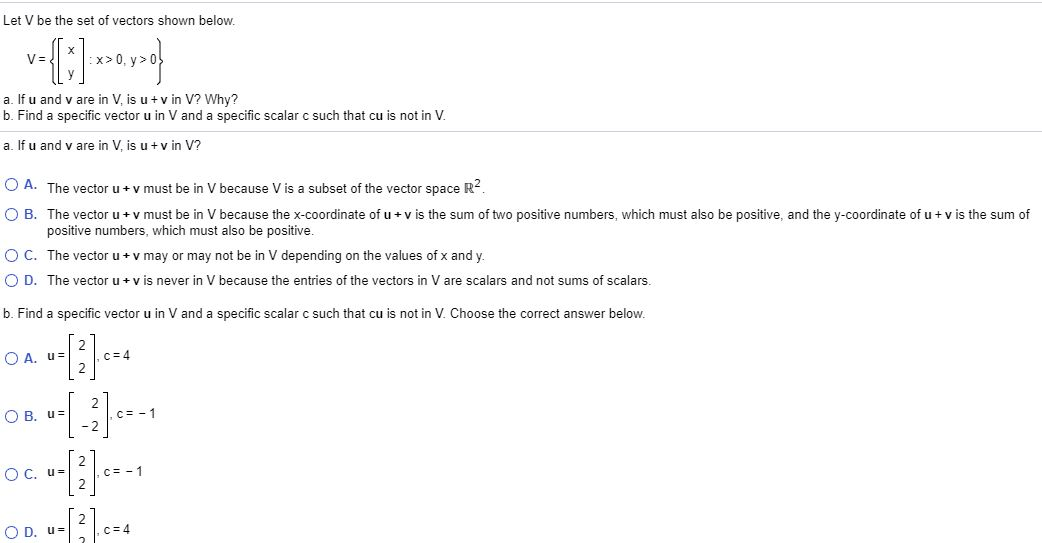Let V be the set of vectors shown below. V= Ox>0, y>0 a. If u and v are in V, is u + v in V? Why? b. Find a specific vector u in V and a specific scalar c such that cu is not in V. a. If u and v are in Vis u + vin V? O A. The vector u + v must be in V because V is a subset of the vector space R2...

• ### Find any vector w that is perpendicular to both vector "u = 3j + 4k" and vector "v = 2i"

Find any vector w that is perpendicular to both vector "u = 3j + 4k" and vector "v = 2i".Note: i, j and k are unit vectorsHow would you solve this problem? Please walk me through?

Free Homework App